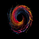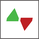# Ehlers Smoothed Adaptive Momentum [LazyBear]

10243 görüntülenme
Bored of Ehlers yet? :) I still have plenty of Ehlers in my collection, was thinking of publishing one of his Fishers or Adaptive RVI next, but @ChartArt requested Smoothed Adaptive Momentum ( SAM ), so here we go...

This is my 200th script (not including the variations and other custom scripts I shared over PM). My complete list of indicators here - http://bit.ly/1LQaPK8.

This smoothed adaptive momentum is straightforward to use (per Ehlers original rules). If it crosses above zero buy the next open, if it crosses below zero sell the next open. Of course, I strongly suggest filtering the signals.

Finally, here's an Ehlers-only chart to help determine where BTC is heading :P

- Ehlers CG Oscillator: - Cybernetic Analysis for Stocks and Futures ( Ehlers )

List of my public indicators: http://bit.ly/1LQaPK8
List of my app-store indicators: http://blog.tradingview.com/?p=970

--Updated chart--

Here's the chart with barcolors ON (forgot to turn it on in the published one)

```//
// @author LazyBear
//
// List of my public indicators: http://bit.ly/1LQaPK8
// List of my app-store indicators: http://blog.tradingview.com/?p=970
//
study("Ehlers Smoothed Adaptive Momentum [LazyBear]", shorttitle="ESAM_LB", overlay=false, precision=3)
src=input(hl2, title="Source")
a=input(.07, title="Alpha")
co=input(8.0, title="Cutoff")
pi=4*atan(1.0), dtr=pi/180.0, rtd=1/dtr
s = (src + 2*src + 2*src + src)/6.0
c = nz(((1 - 0.5*a)*(1 - 0.5*a)*(s - 2*nz(s) + nz(s)) + 2*(1-a)*nz(c) - (1 - a)*(1-a)*nz(c)), (src - 2*src + src)/4.0)
q1 = (.0962*c + 0.5769*nz(c) - 0.5769*nz(c) - .0962*nz(c))*(0.5+.08*nz(ip))
I1 = nz(c)
dp_ = iff(q1 != 0 and q1 != 0, (I1/q1 - nz(I1)/nz(q1)) / (1 + I1*nz(I1)/(q1*nz(q1))),0)
dp = iff(dp_ < 0.1, 0.1, iff(dp_ > 1.1, 1.1, dp_))
med(x,y,z) => (x+y+z) - min(x,min(y,z)) - max(x,max(y,z))
md = med(dp,dp, med(dp, dp, dp))
//dc = iff(md == 0, 15, 6.28318 / md + 0.5)
dc = iff(md == 0, 15, 2*pi / md + 0.5)
ip = .33*dc + .67*nz(ip)
p = .15*ip + .85*nz(p)
pr=round(abs(p-1))
vx0=0
vx1=pr==1?(src - src):vx0
vx2=pr==2?(src - src):vx1
vx3=pr==3?(src - src):vx2
vx4=pr==4?(src - src):vx3
vx5=pr==5?(src - src):vx4
vx6=pr==6?(src - src):vx5
vx7=pr==7?(src - src):vx6
vx8=pr==8?(src - src):vx7
vx9=pr==9?(src - src):vx8
vx10=pr==10?(src - src):vx9
vx11=pr==11?(src - src):vx10
vx12=pr==12?(src - src):vx11
vx13=pr==13?(src - src):vx12
vx14=pr==14?(src - src):vx13
vx15=pr==15?(src - src):vx14
vx16=pr==16?(src - src):vx15
vx17=pr==17?(src - src):vx16
vx18=pr==18?(src - src):vx17
vx19=pr==19?(src - src):vx18
vx20=pr==20?(src - src):vx19
vx21=pr==21?(src - src):vx20
vx22=pr==22?(src - src):vx21
vx23=pr==23?(src - src):vx22
vx24=pr==24?(src - src):vx23
vx25=pr==25?(src - src):vx24
vx26=pr==26?(src - src):vx25
vx27=pr==27?(src - src):vx26
vx28=pr==28?(src - src):vx27
vx29=pr==29?(src - src):vx28
vx30=pr==30?(src - src):vx29
vx31=pr==31?(src - src):vx30
vx32=pr==32?(src - src):vx31
vx33=pr==33?(src - src):vx32
vx34=pr==34?(src - src):vx33
vx35=pr==35?(src - src):vx34
vx36=pr==36?(src - src):vx35
vx37=pr==37?(src - src):vx36
vx38=pr==38?(src - src):vx37
vx39=pr==39?(src - src):vx38
vx40=pr==40?(src - src):vx39
vx41=pr==41?(src - src):vx40
vx42=pr==42?(src - src):vx41
vx43=pr==43?(src - src):vx42
vx44=pr==44?(src - src):vx43
vx45=pr==45?(src - src):vx44
vx46=pr==46?(src - src):vx45
vx47=pr==47?(src - src):vx46
vx48=pr==48?(src - src):vx47
vx49=pr==49?(src - src):vx48
vx50=pr==50?(src - src):vx49
vx51=pr==51?(src - src):vx50
vx52=pr==52?(src - src):vx51
vx53=pr==53?(src - src):vx52
vx54=pr==54?(src - src):vx53
vx55=pr==55?(src - src):vx54
vx56=pr==56?(src - src):vx55
vx57=pr==57?(src - src):vx56
vx58=pr==58?(src - src):vx57
vx59=pr==59?(src - src):vx58
vx60=pr==60?(src - src):vx59
vx61=pr==61?(src - src):vx60
vx62=pr==62?(src - src):vx61
vx63=pr==63?(src - src):vx62
vx64=pr==64?(src - src):vx63
vx65=pr==65?(src - src):vx64
vx66=pr==66?(src - src):vx65
vx67=pr==67?(src - src):vx66
vx68=pr==68?(src - src):vx67
vx69=pr==69?(src - src):vx68
vx70=pr==70?(src - src):vx69
vx71=pr==71?(src - src):vx70
vx72=pr==72?(src - src):vx71
vx73=pr==73?(src - src):vx72
vx74=pr==74?(src - src):vx73
vx75=pr==75?(src - src):vx74
v1=vx75
a1=exp(-pi/co)
b1=2.0*a1*cos((1.738*180/co)*dtr)
c1=a1*a1
coef2=b1+c1
coef3= -(c1+b1*c1)
coef4=c1*c1
coef1=1-coef2-coef3-coef4
f3=nz(coef1*v1+coef2*nz(f3)+coef3*nz(f3)+coef4*nz(f3), v1)
ml=plot(0, color=gray, title="ZeroLine")
pl=plot(f3>0?f3:0,title="DummyP",color=gray, linewidth=0, style=circles)
nl=plot(f3<0?f3:0,title="DummyN",color=gray, linewidth=0, style=circles)
plot(f3, title="Ehlers SAMI", color=black, linewidth=2)
fill(pl, ml, green, title="PositiveFill", transp=70), fill(nl, ml, red, title="NegativeFill", transp=70)
ebc=input(false, title="Enable bar colors")
bc=ebc?(f3>0?f3>nz(f3)?lime:green:f3<f3?red:orange):na
barcolor(bc)```
List of my free indicators: http://bit.ly/1LQaPK8
List of my indicators at Appstore: http://blog.tradingview.com/?p=970

## YorumlarAs always thank you very much for your relentless contributions to Tradingview! I signed up here last year, because I was doing my first baby steps in trading and found on Youtube an interesting video about the 'WaveTrend Oscillator' and then a quick Google search brought me here to your well known WT Tradingview script (https://www.tradingview.com/script/2KE8wTuF-Indicator-WaveTrend-Oscillator-WT/) which now has more than 1500 user favorites. So this is where it all began for me here, as a little anecdote to your 200th published script :)

This indicator, the Ehlers Smoothed Adaptive Momentum, gives a good signal of the dominant (mega)trend of the price. Below is a weekly Bitcoin chart as example, which clearly shows where the overall Bitcoin downtrend began (in July 2014 when GHash.IO made negative newspaper headlines around the world with the 51% mining pool problem, which let well known Bitcoin developer Peter Todd to sell 50% of all of his Bitcoins that month (http://www.reddit.com/r/Bitcoin/comments/281ftd/why_i_just_sold_50_of_my_bitcoins_ghashio/). Yes, that is the month where this Ehlers price momentum indicator also starts to "turn red" signaling a downtrend when I posted one of my first Tradingview charts (https://www.tradingview.com/chart/BTCUSD/wRFOPTbL-Bitcoin-is-facing-a-larger-correction/) sadly without having this indicator - which would have kept me in a very profitable bearish mood all these months since then.

Cevap GönderChartArt